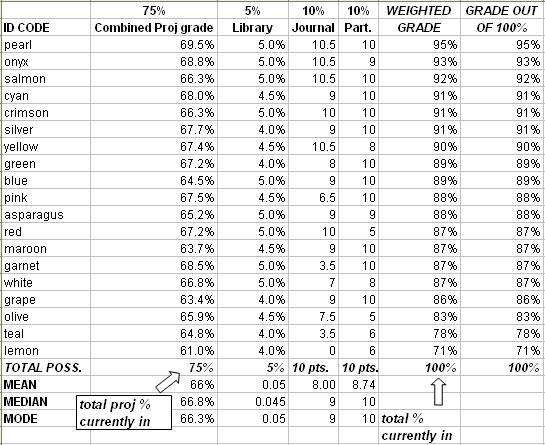# SRS 200Final Grades: Fall 2006

### Explanation of statistics:

Mean: the average
Median: the "middle" score (for our class of 19, 10 are at or above the median score and 10 are at or below the median score.)
Mode: the most common score

Grades are computed on the following scale:

• 93 - 100 = A
• 90 - 92 = A-
• 87 - 89 = B+
• 83 - 86 = B
• 80 - 82 = B-
• 77 - 79 = C+
• 73 - 76 = C
• 70 - 72 = C-
• 60 - 69 = D
• 0 - 59 = F

About the Combined Proj grade column: To ensure anonymity, this column reflects your total grade of ALL projects as a single percentage. The bottom of the column shows the total percentage for the projects that has been turned in thus far (where it says "total proj % currently in"). So if 25% has been turned in so far and your score is 22%, then you are 3% short of the maximum.

About the Journal and Participation score columns: Remember that you are working your way up to 10 points in each of these columns. The total possible points you can currently have appears at the bottom of each of those columns. For participation, you can obtain 1 point per week.

How to use the WEIGHTED GRADE column: This column can tell you the maximum possible score that you can still get in the course. If the total % currently in is, say, 30%, and your score is 18%, then that means you are 12% (30-18) short of the maximum. So your maximum possible score for the entire course is 100-12 = 88% = B+. This assumes you don't lose any more points for the rest of the term (which is unrealistic for any human). So just treat it as a way to figure out what is still mathematically possible.SRS 200 homepage